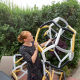# #MathArtChallenge Day 42: Origami Octahedron

The Challenge: Fold you own octahedron from 6 square pieces of paper.

Materials Needed: 6 square pieces of paper.
Math concepts you could explore with this challenge: angles, geometric construction, geometry, origami, symmetry, vertices/intersections

Depending on how you use this activity, you may engage with different mathematical standards. I’ve listed possible connected math content above. Here are a few suggestions for how you might integrate the 8 mathematical practices. Feel free to add your own suggestions in the comments!

2.) Reason abstractly and quantitatively. Why is it that an octahedron (8 faces) can be made from 6 pieces of paper?

4.) Model with mathematics. This makes for a really nice physical model for 3 axis grids. You can help students graph 3D coordinates (x, y, z) using this if you make it from graph paper.## Author: Ms. P

Math Teacher in Minneapolis, MN.

Posted on